WPS Office

Free All-in-One Office Suite with PDF Editor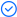Read, edit, and convert PDFs with the powerful PDF toolkit.Microsoft-like interface, easy to use.

Windows • MacOS • Linux • iOS • Android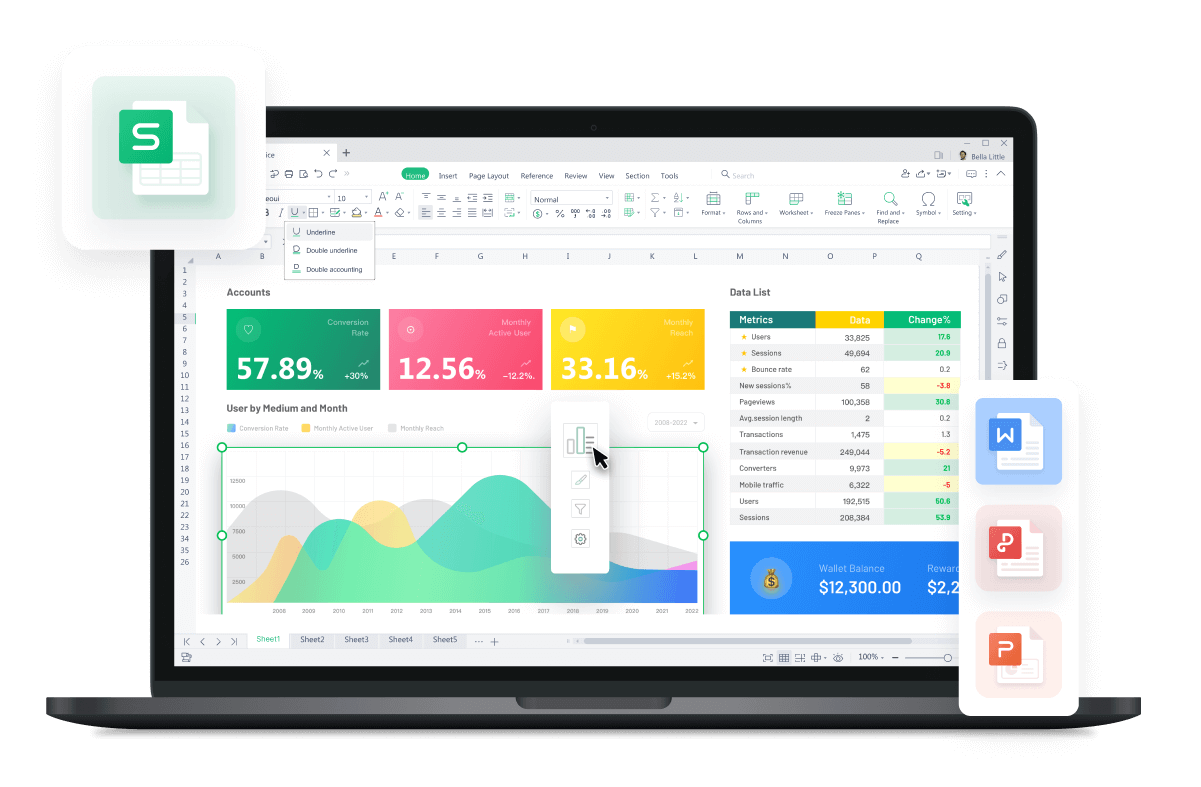# How to Use SUMIF Text in Excel

August 1, 2022
6.0K Views

SUMIF function allows you to sum the values in a range that meets specific criteria that you specify.

Whether you're working with large datasets or need to quickly calculate the sum of a few cells, the SUMIF function can save you time and effort.

This article will show you how to use the SUMIF function easily in Excel. We'll also provide some examples of how the SUMIF function can be used in real-world scenarios. Keep reading!

## SUMIF FUNCTION SYNTAX

The SUMIF function in Excel is a predefined function that computes the sum of elements in a range based on a true or false condition.

It is written as =SUMIF:

The condition is referred to as criteria, which can check things like:

• If a number is more than another number, >

• If a number is less than another number, >

• If a number or text is equal to something, =

• The [sum_range] is the range over which the function calculates the sum.

## How to use the SUMIF function in Excel with dates

In Excel, The SUMIF function is used to sum values within a range that meets certain criteria. The criteria can be a number, text, or date. Users can use the SUMIF function in Excel with dates, this function allows you to calculate the sum of values that meet specific date criteria.

To use the SUMIF function in Excel with dates, you can follow these steps:

• In the cell where you want the sum, enter the SUMIF function.

• Enter a range of cells containing the dates you want to use as criteria in the range argument.

• In the criteria argument, specify the date you want to use as the criteria. You can either refer to a cell that contains the date or input the date directly into the function.

• In the sum_range argument, specify the range of cells that contain the values you want to sum.

### Example

Suppose you have a table that contains dates in column A and sales in column B, and you want to sum the sales in the month of January.

Here's how you can use the SUMIF function:

• Type “=SUMIF” in a blank cell where you want the total sales to be generated for your range.

• Select the range of cells with dates; “A2:A25”

• Type down the criteria; “

• Lastly, select the range of cells that contain Sales; B2:B25

• Press enter for the results.

### Pros of Excel functions with dates

• Excel functions with dates enable you to analyze and visualize data in a variety of ways.

• Excel date functions allow you to produce professional-looking reports and presentations.

### Cons of Excel functions with dates

• Excel may have limits when working with extremely large datasets or complex date-based calculations.

• While Excel has a variety of date capabilities, it may not be appropriate for all types of calculations or jobs.

## How to use the SUMIF function in Excel with multiple sheets

Excel functions that work with multiple sheets allow users to perform calculations and operations across multiple worksheets or workbooks, allowing for efficient data analysis and organization. These functions are especially helpful when working with big amounts of data distributed over multiple sheets or workbooks.

Excel offers various functions that support calculations involving multiple sheets, but today we will be learning how to use the SUMIF Function in Excel with multiple sheets. The SUMIF Function allows users to reference cells, ranges, or values from different sheets by specifying the sheet name along with the cell or range reference.

To use the SUMIF function in Excel with dates, you can follow these steps:

• Go to the sheet and select the cell where you want the sum, enter the SUMIF function.

• Enter a range of cells from the sheet containing the dates you want to use as criteria in the range argument.

• In the criteria argument, specify the date you want to use as the criteria. You can either refer to a cell that contains the date or input the date directly into the function.

• In the sum_range argument, specify the SUMIF excel data range of cells that contain the values you want to sum.

• The following steps need to be repeated for each sheet.

### Example

Suppose you have a workbook with three sheets named US, UK, and Canada. Each sheet contains sales data for a different region, and you want to sum the sales figures for a specific month across all three regions. Here's how you can do it:

• Open the workbook that contains all the sales records.

• Type the SUMIF function in the cell where results are required.

• The formula will start from the “US sales” sheet, select the range; A3:A26.

• Type down the criteria; “

• Select the sum range; B3:B26.

• Add a “+” and continue with the SUMIF formula for UK and Canada Sales.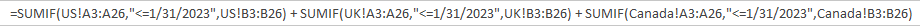• Press enter for results.

### Pros of Excel functions with multiple sheets

• Excel functions with multiple sheets provide a high level of customization, allowing users to design and structure their data.

• Wide range of applications.

### Cons of Excel functions with multiple sheets

• Difficulty in viewing all data on a single page.

## How to use the SUMIF function in Excel with multiple criteria

When working with Excel, the SUMIF function can be a powerful tool for performing calculations and operations based on multiple criteria. With this function, users can filter and analyze data using more than one condition or criteria, providing greater flexibility and accuracy in data analysis.

The ability to combine multiple criteria using logical operators like AND, OR, and NOT further enhances the functionality and versatility of Excel. This multifunctional capability allows for efficient data analysis and the creation of complex conditions to meet specific data analysis requirements.

Here is how SUMIF Function multiple criteria can be utilized:

• Identify the range of cells containing the values you want to sum and the range of cells containing the criteria.

• Determine the conditions or criteria you want to apply. You can use logical operators such as ">,

=, , =" to define your criteria.

• Set up a formula in a cell where you want the result to appear.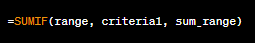• Replace "range" with the range of cells containing the criteria to evaluate.

• Replace "criteria1" with the first condition you want to apply.

• Replace "sum_range" with the range of cells containing the values to be summed.

• If you have additional criteria, use the SUMIFS function instead. The syntax is similar, but you can specify multiple conditions.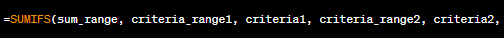• Press Enter to calculate the sum based on the specified criteria.

### Example

Let's say we want to calculate the total sales for the US region in January where the sales are greater than \$250.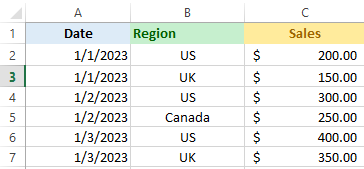• Select a cell where you want the result to appear. For example, let's choose cell G4.

• We will be using “SUMIFS” function for setting multiple criteria

• Enter “=SUMIFS(“ and select the range of data; C2:C7. This command will tell excel to sum the values in cells C1:C7.

• We will then set our first criteria; the dates should be greater than 1st January and less than 31st January. Pro tip: Define the data type “&date”. Here is how the formula should look like:• We will not select our second criteria; our sales should be from the “US” region only. This will command excel to sum values of sales in the US region only.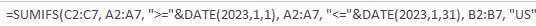• Our last criteria is to calculate the sales which are above \$250 only, therefore we will set up the last criteria as well.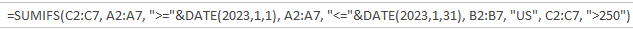• The final result shows the Sum of Sales in the month of January for US that were above \$250.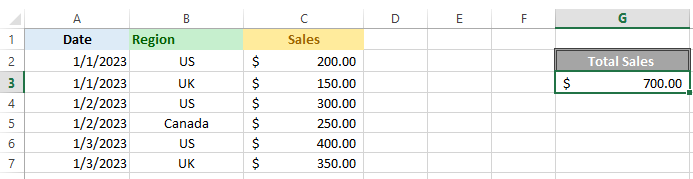### Pros of Excel functions with multiple criteria

• Complex situations require logical operators such as AND, OR, NOT.

• Integration with other Excel functions such as COUNTIF and IF-THEN.

### Cons of Excel functions with multiple criteria

• When compared to working with a single sheet, working with many sheets may involve more processes and complexity.

• Using Excel functions with many sheets efficiently may necessitate some amount of experience.

## Troubleshooting and Tips

### Why is the SUMIF function not working properly?

There could be several reasons why the SUMIF function may not be working properly. Here are a few common issues to consider:

• Incorrect syntax

• Data format mismatch

• Trailing spaces or hidden characters

• Error values or non-numeric data

• Multiple criteria logic

• Cell references

### What is the correct way to apply the Sumif formula?

• The right way to use the SUMIF formula in Excel is as follows:

• Determine the range of cells containing the values to be summed.

• Determine the range of cells that contain the desired criteria.

• Create the SUMIF function and specify the range of cells containing the criteria, the criteria itself, and the range of values to sum.

Here is the SUMIF function Syntax for reference:

=SUMIF(range, criteria, [sum_range])

## FAQ

### Why is my sum formula not accurate in Excel?

There could be various reasons why your SUM formula in Excel is inaccurate. Consider the following possible causes:

• Opening closing brackets should be matched, the number of brackets opening should be equal to the number of brackets closing

• Incorrect Range

• Mixed Data Types

• Not following the syntax correctly; range, criteria and sum_range need to be correctly entered in the same order.

• Make sure you’re not nesting more 64 functions

• Make sure numbers are entered without formatting

### How do you use the Averageif function?

AverageIF function is a built-in excel function and can be used by simply entering “=AverageIF” in an empty cell. The following has to be followed to successfully execute the AverageIF function on excel:

=AVERAGEIF(range, criteria, [average_range])

### Can I use multiple criteria with the SUMIF function in Excel?

Yes, you can use multiple criteria with the SUMIF function in Excel by using the SUMIFS function instead. The SUMIFS function allows you to sum the values in a range of cells that meet multiple criteria or conditions.

## Summary

Throughout the article, we have covered various aspects of the SUMIF function, including its syntax, usage with dates, multiple sheets, and multiple criteria using logical operators. By following the provided steps and examples, users can effectively utilize the SUMIF function to perform calculations and analyze data.

Furthermore, users can also leverage WPS Spreadsheets, a powerful alternative to Excel, to achieve the same functionality and effective results. WPS Spreadsheets offers a user-friendly interface and extensive features that enable users to perform data analysis and calculations effortlessly. It supports the SUMIF function and other Excel functions, ensuring compatibility and ease of use for those transitioning from Excel. By utilizing WPS Spreadsheets, users can confidently work with data, perform calculations, and generate valuable insights. Interested to learn more about WPS Spreadsheets? Visit WPS official website now to explore their office suite.